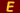# The Ecphorizer

 Le Coin d'Archimede 2 Nikolai Ivanovich Lobachevski

#### Issue #10 (June 1982)

Spring has finally arrived, and for many of us it is the time we start those building projects we put off all winter. One of our wealthy (but very thrifty) local members had the good fortune to be able to buy a neat lake which oddly enough was in the shape of a triangle: 14 miles on one side, 15 miles on another, and 13 miles on the third side. She decided to build a summer home for herself on one side and summer homes for each of her two children on each of the other two sides, so that she could visit her daughter, then her son, and then return home using the least amount of gasoline possible in her motorboat. Where should she build these three homes; can you pinpoint the locations exactly?

Question from an "IQ Exam": What number is as much more than 20 as it is less than one half of what 70 is 10 less than? Who writes this crazy stuff, anyway?

Last Month's Solution. The Sun God was well supplied with cattle. If:

A = black bulls B = spotted bulls C = brown bulls D white bulls E = white cows F = black cows G = spotted cows H = brown cows, D= (l/2+l/3)A+C (5/6)A+C;

A = (1/4 + 1/5)B + C = (9/20)B + C; and B = (13/42)D + C. Solving A, B, D in terms of C,

A = (178/99)C, B = (l580/891)C, and D = (742/297)C. For cows, E (1/3+1/4) (A+F);

F = (9/20) (B+G); G = (11/30) (C+H); and

C = (13/42) (D+E).

Solve for any of the above in terms of D, A, B, C; e.g. 4,657 E = 2,800 A + 1,260 B + 462 C + 143 D.

Substituting the values obtained above for D, A, B in terms of C yields the following ratio: E/C = 7,206,360/4,149,387. Similarly, the ratios F/C, G/C, and H/C may be calculated. 4,149,387 = 4,657 x 891; since 4,657 is prime and 891 is the least value for an integral solution, the quantities are as follows, where (n) may be 1, 2, 3,... etc.

 n=n Bulls Cows Black A "7,460,514 (n)" F "4,893,246 (n)" Spotted B "7,358,060 (n)" G "3,515,820 (n)" Brown C "4,149,387 (n)" H "5,439,21 (n)" White D "10,366,482 (n)" E "7,206,360 (n)"While working on this month's puzzle, he was heard to hum, "plagiarize, let no one else's work evade you eyes, remember why the good Lord made your eyes, so plagiarize, plagiarize, plagiarize - only be sure always to call it please "research."

More Articles by Nikolai Ivanovich Lobachevski

close
Title: